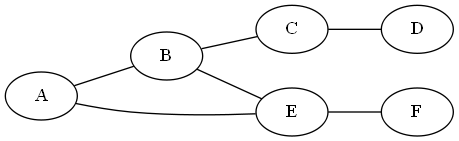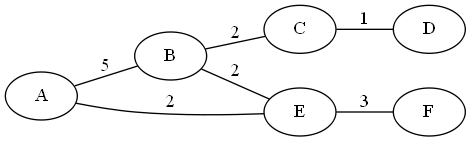# Tag Archives: 3-Partition

## Weighted Diameter

It took over a year (the first problem strictly from the Appendix was Domatic Number, last August), but we’re finally at the end of the Graph Theory section!  And the last problem is one that’s actually good for students to solve.

The problem: Weighted Diameter.  This is problem GT65 in the appendix

The description: Given a graph G=(V,E) a collection C of  |E| not necessarily distinct, non-negative integers, and a positive integer K.  Can we find a one-to-one function f  mapping each edge in E to an element of C such that if f(e) is the length of edge e, then G has a diameter of K or less.

In other words, given a set of edge weights C, can we give each edge in E a (distinct) weight from C such that the resulting weighted graph has a path between any two vertices of length ≤ K?

Example: Suppose I have a graph:And C= {1,2,2,2,3,5}.  I think the best was you can label this is:The Diameter here is 7 (The length of the path from A-D).

The reduction: G&J say to use 3-Partition, so we’ll go with that.  We’re given a set A, with 3m elements, a bound B, and want to split the elements of B into sets of size 3 so that each set adds up to m.  We know several things about A, but the important thing for our purposes is that all of the elements in A add up to m*B.

We’ll also assume that |A| is at least 9.  If it’s smaller than that, we can just brute-force the answer.

What we’re going to do is build a graph that is a tree with 3m+1 vertices.  We have a root, and the root has m chains of length 3 extending from it. This gives us exactly 3*m edges.

We set K = 2*B, and set C = A

If there exists a 3-partition of A, then each of the sets of 3 elements can map onto a different chain in the graph.  This makes the longest path in the graph be between any 2 leaves.  Since the length from a leaf to a root is exactly B, the diameter of the graph is 2B.

If there exists a weighted diameter of the graph of cost 2*B, then we need to show that the cost of each chain is exactly B.  Suppose it wasn’t, and the cost from the root to some leaf v is > B, let’s say B+x.  Then, since there are at least 3 chains (since |A| >= 9) and since the sum of all of the weights is m*B exactly), there must exist some leaf w, with the cost of the chain from the root to w > B-x.  The cost of the path from v to w is now > 2B, a contradiction.

If the cost from the root to some leaf is < B, then there must be some other leaf u with the cost from the root to u > B (since the costs of all of the edges add up to m*B), and we can do the above on u.

Since each chain costs exactly B, we can use the edge weights of each chain as the sets of 3 elements that make our 3-Partition.

Difficulty: 4. G&J does say in the comments that this problem is NP-Complete even for trees, so that may have been a hint.  The proof is a little tricky (getting from “diameter ≤ K” to “set adding up to exactly K” requires some work, and there may be a more elegant way than what I did).  But I think this would make a good homework problem.